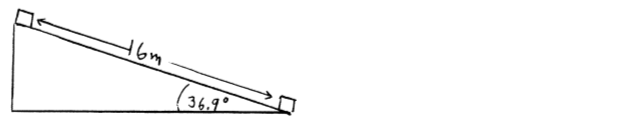Problem: A block of mass 10.0 kg slides 16.0 m down a 36.9° incline, from point A at the top of the incline to point B at the bottom. As the block moves from point A to point B, the surface of the incline exerts a constant friction force that has magnitude 42.0 N.As the block moves from A to B, how much work is done on it by the friction force? (Be sure to indicate whether the work is positive or negative).

FREE Expert Solution
90% (435 ratings)
Problem Details

A block of mass 10.0 kg slides 16.0 m down a 36.9° incline, from point A at the top of the incline to point B at the bottom. As the block moves from point A to point B, the surface of the incline exerts a constant friction force that has magnitude 42.0 N.

As the block moves from A to B, how much work is done on it by the friction force? (Be sure to indicate whether the work is positive or negative).